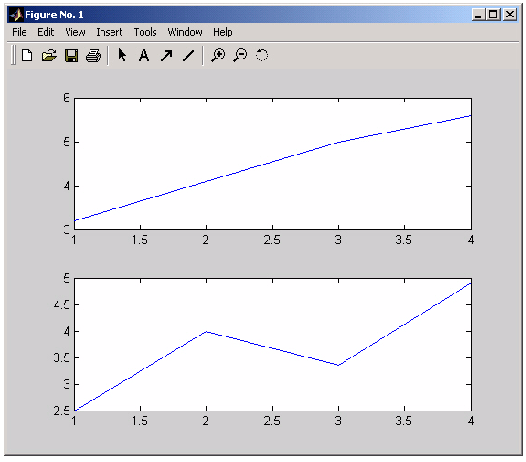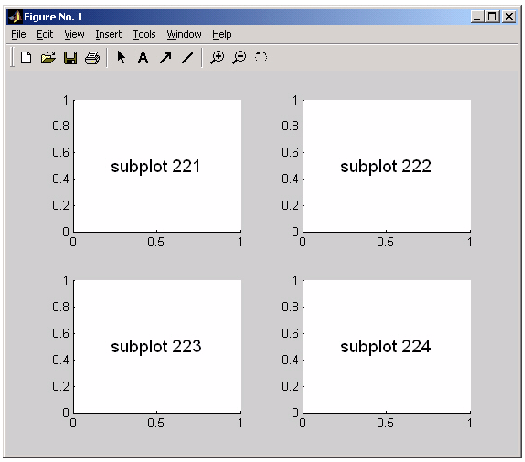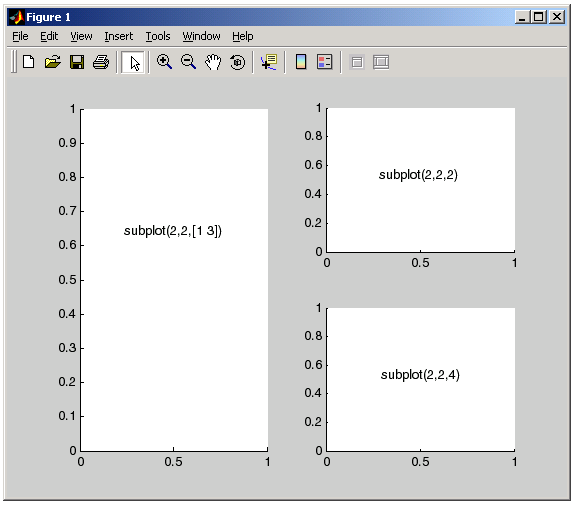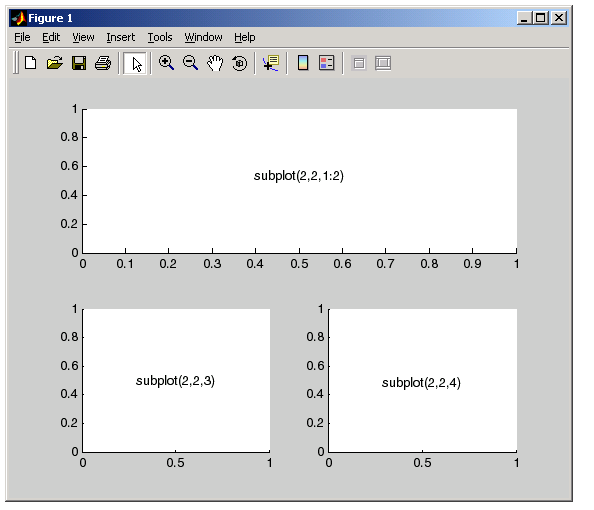MATLAB Function Referencesubplot

Create axes object in tiled positions

Syntax

• ```subplot(m`,`n`,`p)
subplot(mnp)
subplot(m,n,p,'replace')
subplot(m,n,p,'align')
subplot(h)
subplot('Position',[left bottom width height])
h = subplot(...)
```

Description

`subplot` divides the current figure into rectangular panes that are numbered rowwise. Each pane contains an axes object. Subsequent plots are output to the current pane.

`h = subplot(m,n,p)`, or `subplot(mnp)` breaks the Figure window into an `m`-by-`n` matrix of small axes, selects the `p`th axes object for for the current plot, and returns the axis handle. The axes are counted along the top row of the Figure window, then the second row, etc. For example,

• ````subplot`(2,1,1), `plot`(income)
`subplot`(2,1,2), `plot`(outgo)
```

plots income on the top half of the window and outgo on the bottom half. If the `CurrentAxes` is nested in a uipanel, the panel is used as the parent for the subplot instead of the current figure. The new axes object becomes the current axes.

If `p` is a vector, it specifies an axes object having a position that covers all the subplot positions listed in `p`.

```subplot(m,n,p,'replace') ``` If the specified axes object already exists, delete it and create a new axes.

```subplot(m,n,p,'align') ``` positions the individual axes so that the plot boxes align, but does not prevent the labels and ticks from overlapping.

```subplot(h) ``` makes the axes object with handle `h` current for subsequent plotting commands.

```subplot('Position',[left bottom width height]) ``` creates an axes at the position specified by a four-element vector. `left`, `bottom`, `width`, and `height` are in normalized coordinates in the range from 0.0 to 1.0.

```h = subplot(...) ``` returns the handle to the new axes object.

Remarks

If a `subplot` specification causes a new axes object to overlap any existing axes, then `subplot` deletes the existing axes object and uicontrol objects. However, if the `subplot` specification exactly matches the position of an existing axes object, then the matching axes object is not deleted and it becomes the current axes.

`subplot(1,1,1)` or `clf` deletes all axes objects and returns to the default `subplot(1,1,1)` configuration.

You can omit the parentheses and specify subplot as

• ```subplot mnp
```

where `m` refers to the row, `n` refers to the column, and `p` specifies the pane.

Special Case - subplot(111)

The command `subplot(111)` is not identical in behavior to `subplot(1,1,1)` and exists only for compatibility with previous releases. This syntax does not immediately create an axes object, but instead sets up the figure so that the next graphics command executes a `clf`` reset` (deleting all figure children) and creates a new axes object in the default position. This syntax does not return a handle, so it is an error to specify a return argument. (This behavior is implemented by setting the figure's `NextPlot` property to `replace`.)

Examples

To plot `income` in the top half of a figure and `outgo` in the bottom half,

• ```income = [3.2 4.1 5.0 5.6];
outgo = [2.5 4.0 3.35 4.9];
subplot(2`,`1`,`1); plot(income)
subplot(2`,`1`,`2); plot(outgo)```

The following illustration shows four subplot regions and indicates the command used to create each.The following combinations produce asymmetrical arrangements of subplots.

• ```subplot(2,2,[1 3])
subplot(2,2,2)
subplot(2,2,4)
```You can also use the colon operator to specify multiple locations if they are in sequence.

• ```subplot(2,2,1:2)
subplot(2,2,3)
subplot(2,2,4)```

`axes`, `cla`, `clf`, `figure`, `gca`